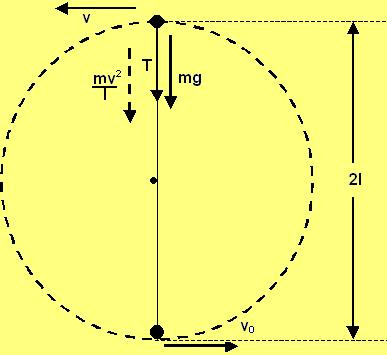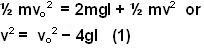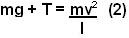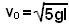## Saturday, May 3, 2008

### Irodov Problem 1.137Suppose we impart a velocity vo
at the bottom. As the particle moves up it gains potential energy and looses its kinetic energy i.e. velocity. Suppose that at the top-most point its velocity is v. By the time the particle reaches the top-most point it has gained a height of 2l and hence a potential energy of 2mgl. From conservation of energy we have,In order for the particle to complete the circle at no point during the particles motion there should be any slack in the string, i.e. it should be tense at all points.

There are two forces acting on the particle at the top-most point, i) the force of gravity mg and ii) the tension in the string both acting to pull the particle down. Since the particle is rotating in a circle of radius l , it is subject to a centripetal acceleration of. Hence we have,The minimum value of vo corresponds to the case when at the top-most point the tension T is exactly 0, i.e. the particle just makes it in the loop. Substituting this condition we have,From (3) and (1) we have,When the particle is in the horizontal position its potential energy gained with respect to the lowermost position is mgl since its at a height l. Let its speed by v1 then we have,At the horizontal position there is only one force acting in the horizontal direction - the tension in the string T. The particle is also subject to centripetal acceleration by the virtue of its motion in a circle of radius l. So we have,